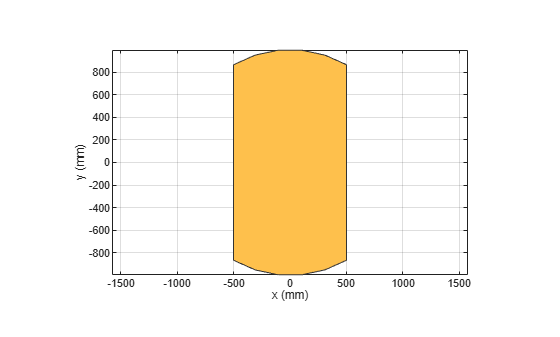# and

Shape1 & Shape2

## Syntax

``c = and(shape1,shape2)``

## Description

example

````c = and(shape1,shape2)` calls the syntax `shape1 & shape2` to intersect two shapes.```

## Examples

collapse all

```Create a rectangular and circular shape and intersect them. ```
```r = antenna.Rectangle; c = antenna.Circle; r&c;```## Input Arguments

collapse all

Shapes created using custom elements and shape objects of Antenna Toolbox™, specified as an object.

Example: `rectangle1&rectangle2` where rectangle1 and rectangle2 are shapes created using `antenna.Rectangle` object.

## Version History

Introduced in R2017a